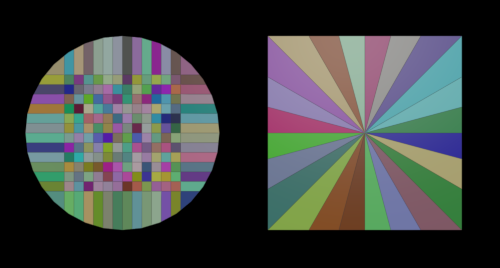## Recommended Posts

Here are two simple clipping tools using python SOP verbs: Bricker and Radial.Unlike the divide SOP it´s cutting always right through the center.

Edited by konstantin magnus
•6

##### Share on other sites

Some exemplary code for easy access:

```import math
node = hou.pwd()
geo = node.geometry()

cuts = node.evalParm('cuts')

clip = hou.sopNodeTypeCategory().nodeVerb('clip')

for i in range(cuts - 1):
angle = i / (cuts - 1.0)
dir = hou.Vector3(math.sin(angle * math.pi), 0.0, math.cos(angle * math.pi))
clip.setParms({
'dir': dir,
'clipop': 2
})
geo.execute(clip, [])
if hou.updateProgressAndCheckForInterrupt():
break

node.geometry().clear()
node.geometry().merge(geo)```

•1

## Create an account

Register a new account